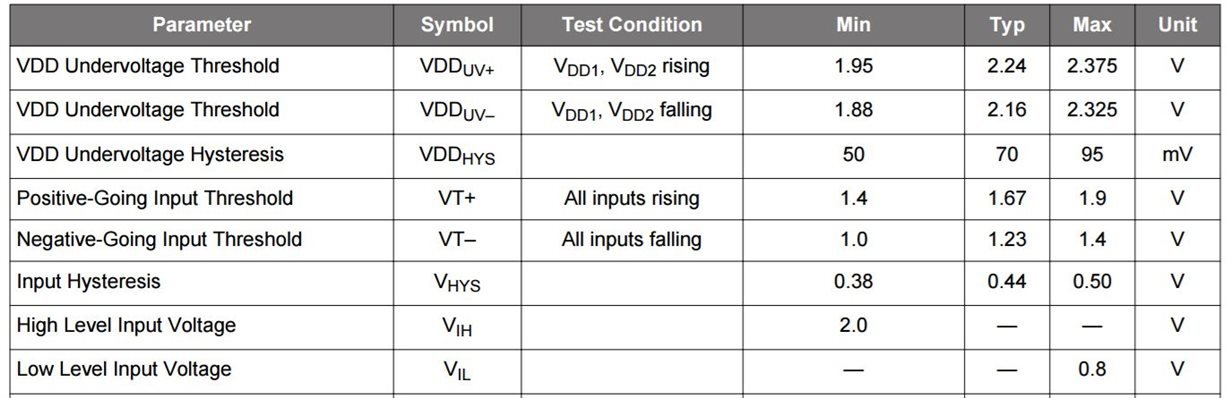If you have a related question, please click the "Ask a related question" button in the top right corner. The newly created question will be automatically linked to this question.

# ISO7321C: Rising edge threshold and falling edge threshold for INx

Part Number: ISO7321C

Hi Team,

In Silicon Lab datasheet, e.g. SI86xx, the threshold is defined as VT+ 1.67V(typ) and VT- 1.23V(typ).

Do we have the specifications for ISO7321? These specs are required for input voltage tolerant test.Thanks.

Zhou

• Hi Zhou,

It is not specified in the ISO7321 datasheet but we can calculate the range from VIH and VIL parameters.

VT+ and VT- would range between 0.8V and 2.0V. There would also be a typical hysteresis of 460mV at 5V supply operation. Calculating using the same would give you the below table.

 Minimum Maximum VT+ 1.26 2.0 VT- 0.8 1.54

Hope this helps.

Regards,
Anand Reghunathan

• Hi Anand,

I can see a overlap from 1.26V to 1.54V by calculation. The logic is not correct in cross-region.

Do we have design value or test value to show?
Do we have typical value?

Thanks.
Zhou
• Hi Zhou,

There will always be a guaranteed hysteresis of typical value 460mV. (VT+) = (VT-) + 460mV
So when VT+ is at its minimum value, VT- will also be at its minimum value. Similarly for the maximum values also.
This will ensure that there will be no overlap happening over the full range.

Example:
When VT- is 1.5V, VT+ will typically be 460mV higher. (VT+) = 1.96V.
When VT+ is 1.3V, VT- will typically be 460mV lower. (VT+) = 0.84V.

They typical value should fall near the average of min and max but I can't give it an exact value.

Regards,
Anand Reghunathan